The AUTOREG Procedure

## Example 8.5 Money Demand Model

This example estimates the log-log money demand equation by using the maximum likelihood method. The money demand model contains four explanatory variables. The lagged nominal money stock M1 is divided by the current price level GDF to calculate a new variable M1CP since the money stock is assumed to follow the partial adjustment process. The variable M1CP is then used to estimate the coefficient of adjustment. All variables are transformed using the natural logarithm with a DATA step. Refer to Balke and Gordon (1986) for a data description.

The first eight observations are printed using the PRINT procedure and are shown in Output 8.5.1. Note that the first observation of the variables M1CP and INFR are missing. Therefore, the money demand equation is estimated for the period 1968:2 to 1983:4 since PROC AUTOREG ignores the first missing observation. The DATA step that follows generates the transformed variables.

```data money;
date = intnx( 'qtr', '01jan1968'd, _n_-1 );
format date yyqc6.;
input m1 gnp gdf ycb @@;
m = log( 100 * m1 / gdf );
m1cp = log( 100 * lag(m1) / gdf );
y = log( gnp );
intr = log( ycb );
infr = 100 * log( gdf / lag(gdf) );
label m    = 'Real Money Stock (M1)'
m1cp = 'Lagged M1/Current GDF'
y    = 'Real GNP'
intr = 'Yield on Corporate Bonds'
infr = 'Rate of Prices Changes';
datalines;

... more lines ...

```

Output 8.5.1 Money Demand Data Series – First 8 Observations
 Predicted Values and Confidence Limits

Obs date m1 gnp gdf ycb m m1cp y intr infr
1 1968:1 187.15 1036.22 81.18 6.84 5.44041 . 6.94333 1.92279 .
2 1968:2 190.63 1056.02 82.12 6.97 5.44732 5.42890 6.96226 1.94162 1.15127
3 1968:3 194.30 1068.72 82.80 6.98 5.45815 5.43908 6.97422 1.94305 0.82465
4 1968:4 198.55 1071.28 84.04 6.84 5.46492 5.44328 6.97661 1.92279 1.48648
5 1969:1 201.73 1084.15 84.97 7.32 5.46980 5.45391 6.98855 1.99061 1.10054
6 1969:2 203.18 1088.73 86.10 7.54 5.46375 5.45659 6.99277 2.02022 1.32112
7 1969:3 204.18 1091.90 87.49 7.70 5.45265 5.44774 6.99567 2.04122 1.60151
8 1969:4 206.10 1085.53 88.62 8.22 5.44917 5.43981 6.98982 2.10657 1.28331

The money demand equation is first estimated using OLS. The DW=4 option produces generalized Durbin-Watson statistics up to the fourth order. Their exact marginal probabilities (p-values) are also calculated with the DWPROB option. The Durbin-Watson test indicates positive first-order autocorrelation at, say, the 10% confidence level. You can use the Durbin-Watson table, which is available only for 1% and 5% significance points. The relevant upper (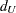) and lower (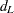) bounds are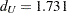and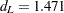, respectively, at 5% significance level. However, the bounds test is inconvenient, since sometimes you may get the statistic in the inconclusive region while the interval between the upper and lower bounds becomes smaller with the increasing sample size. The PROC step follows:

```title 'Partial Adjustment Money Demand Equation';
title2 'Quarterly Data - 1968:2 to 1983:4';

proc autoreg data=money outest=est covout;
model m = m1cp y intr infr / dw=4 dwprob;
run;
```

Output 8.5.2 OLS Estimation of the Partial Adjustment Money Demand Equation
 Partial Adjustment Money Demand Equation Quarterly Data - 1968:2 to 1983:4

The AUTOREG Procedure

Dependent Variable m Real Money Stock (M1)

Ordinary Least Squares Estimates
SSE 0.00271902 DFE 58
MSE 0.0000469 Root MSE 0.00685
SBC -433.68709 AIC -444.40276
MAE 0.00483389 AICC -443.35013
MAPE 0.08888324 HQC -440.18824
Regress R-Square 0.9546
Total R-Square 0.9546

Durbin-Watson Statistics
Order DW Pr < DW Pr > DW
1 1.7355 0.0607 0.9393
2 2.1058 0.5519 0.4481
3 2.0286 0.5002 0.4998
4 2.2835 0.8880 0.1120

 Note: PrDW is the p-value for testing negative autocorrelation.

Parameter Estimates
Variable DF Estimate Standard Error t Value Approx
Pr > |t|
Variable Label
Intercept 1 0.3084 0.2359 1.31 0.1963
m1cp 1 0.8952 0.0439 20.38 <.0001 Lagged M1/Current GDF
y 1 0.0476 0.0122 3.89 0.0003 Real GNP
intr 1 -0.0238 0.007933 -3.00 0.0040 Yield on Corporate Bonds
infr 1 -0.005646 0.001584 -3.56 0.0007 Rate of Prices Changes

The autoregressive model is estimated using the maximum likelihood method. Though the Durbin-Watson test statistic is calculated after correcting the autocorrelation, it should be used with care since the test based on this statistic is not justified theoretically. The PROC step follows:

```proc autoreg data=money;
model m = m1cp y intr infr / nlag=1 method=ml maxit=50;
output out=a p=p pm=pm r=r rm=rm ucl=ucl lcl=lcl
uclm=uclm lclm=lclm;
run;
```
```proc print data=a(obs=8);
var p pm r rm ucl lcl uclm lclm;
run;
```

A difference is shown between the OLS estimates in Output 8.5.2 and the AR(1)-ML estimates in Output 8.5.3. The estimated autocorrelation coefficient is significantly negative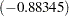. Note that the negative coefficient of AR(1) should be interpreted as a positive autocorrelation.

Two predicted values are produced: predicted values computed for the structural model and predicted values computed for the full model. The full model includes both the structural and error-process parts. The predicted values and residuals are stored in the output data set A, as are the upper and lower 95% confidence limits for the predicted values. Part of the data set A is shown in Output 8.5.4. The first observation is missing since the explanatory variables, M1CP and INFR, are missing for the corresponding observation.

Output 8.5.3 Estimated Partial Adjustment Money Demand Equation
 Partial Adjustment Money Demand Equation Quarterly Data - 1968:2 to 1983:4

The AUTOREG Procedure

Estimates of Autoregressive Parameters
Lag Coefficient Standard Error t Value
1 -0.126273 0.131393 -0.96

 Algorithm converged.

Maximum Likelihood Estimates
SSE 0.00226719 DFE 57
MSE 0.0000398 Root MSE 0.00631
SBC -439.47665 AIC -452.33545
MAE 0.00506044 AICC -450.83545
MAPE 0.09302277 HQC -447.27802
Durbin-Watson 2.1778 Regress R-Square 0.6954
Total R-Square 0.9621

Parameter Estimates
Variable DF Estimate Standard Error t Value Approx
Pr > |t|
Variable Label
Intercept 1 2.4121 0.4880 4.94 <.0001
m1cp 1 0.4086 0.0908 4.50 <.0001 Lagged M1/Current GDF
y 1 0.1509 0.0411 3.67 0.0005 Real GNP
intr 1 -0.1101 0.0159 -6.92 <.0001 Yield on Corporate Bonds
infr 1 -0.006348 0.001834 -3.46 0.0010 Rate of Prices Changes
AR1 1 -0.8835 0.0686 -12.89 <.0001

Autoregressive parameters assumed given
Variable DF Estimate Standard Error t Value Approx
Pr > |t|
Variable Label
Intercept 1 2.4121 0.4685 5.15 <.0001
m1cp 1 0.4086 0.0840 4.87 <.0001 Lagged M1/Current GDF
y 1 0.1509 0.0402 3.75 0.0004 Real GNP
intr 1 -0.1101 0.0155 -7.08 <.0001 Yield on Corporate Bonds
infr 1 -0.006348 0.001828 -3.47 0.0010 Rate of Prices Changes

Output 8.5.4 Partial List of the Predicted Values
 Partial Adjustment Money Demand Equation Quarterly Data - 1968:2 to 1983:4

Obs p pm r rm ucl lcl uclm lclm
1 . . . . . . . .
2 5.45962 5.45962 -.005763043 -0.012301 5.49319 5.42606 5.47962 5.43962
3 5.45663 5.46750 0.001511258 -0.009356 5.47987 5.43340 5.48700 5.44800
4 5.45934 5.46761 0.005574104 -0.002691 5.48267 5.43601 5.48723 5.44799
5 5.46636 5.46874 0.003442075 0.001064 5.48903 5.44369 5.48757 5.44991
6 5.46675 5.46581 -.002994443 -0.002054 5.48925 5.44424 5.48444 5.44718
7 5.45672 5.45854 -.004074196 -0.005889 5.47882 5.43462 5.47667 5.44040
8 5.44404 5.44924 0.005136019 -0.000066 5.46604 5.42203 5.46726 5.43122Previous Page | Next Page | Top of Page